Question

# Question 1 of 7 (1 point) Attempt 1 of 1 View question in a pour och...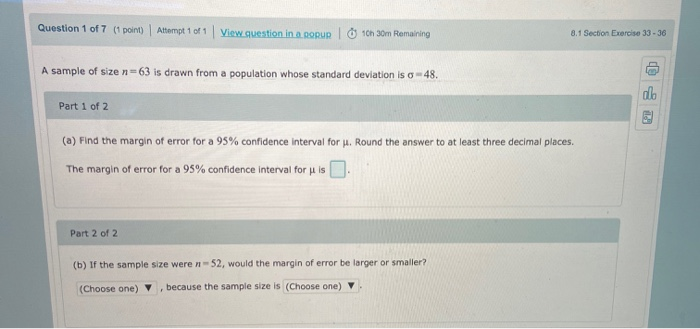Question 1 of 7 (1 point) Attempt 1 of 1 View question in a pour och 30m Remaining 8.1 Section Exercise 33-36 A sample of size n=63 is drawn from a population whose standard deviation is o - 48. co Part 1 of 2 (a) Find the margin of error for a 95% confidence interval for J. Round the answer to at least three decimal places. The margin of error for a 95% confidence interval for u is Part 2 of 2 (b) If the sample size were n-52, would the margin of error be larger or smaller? (Choose one) because the sample size is (Choose one)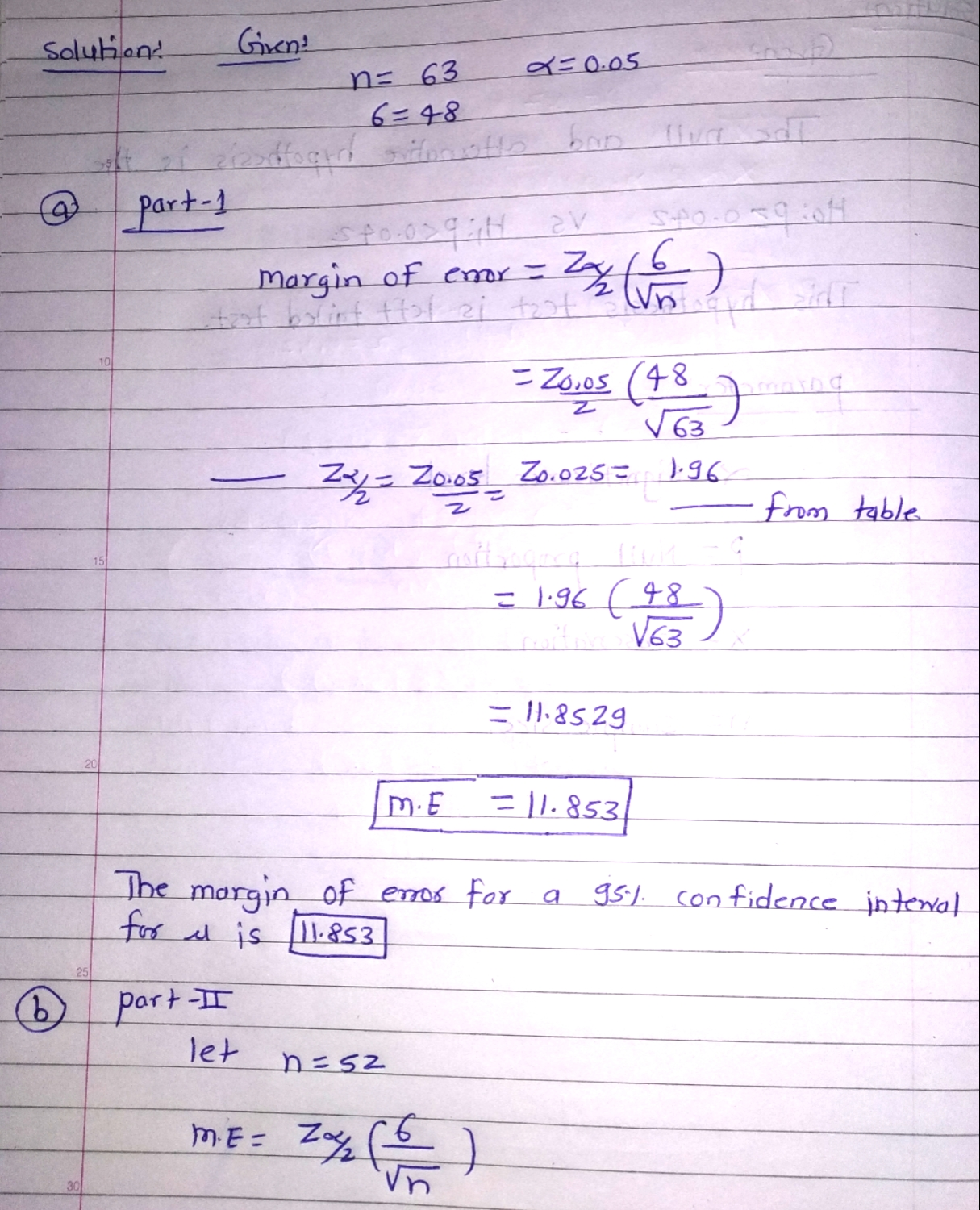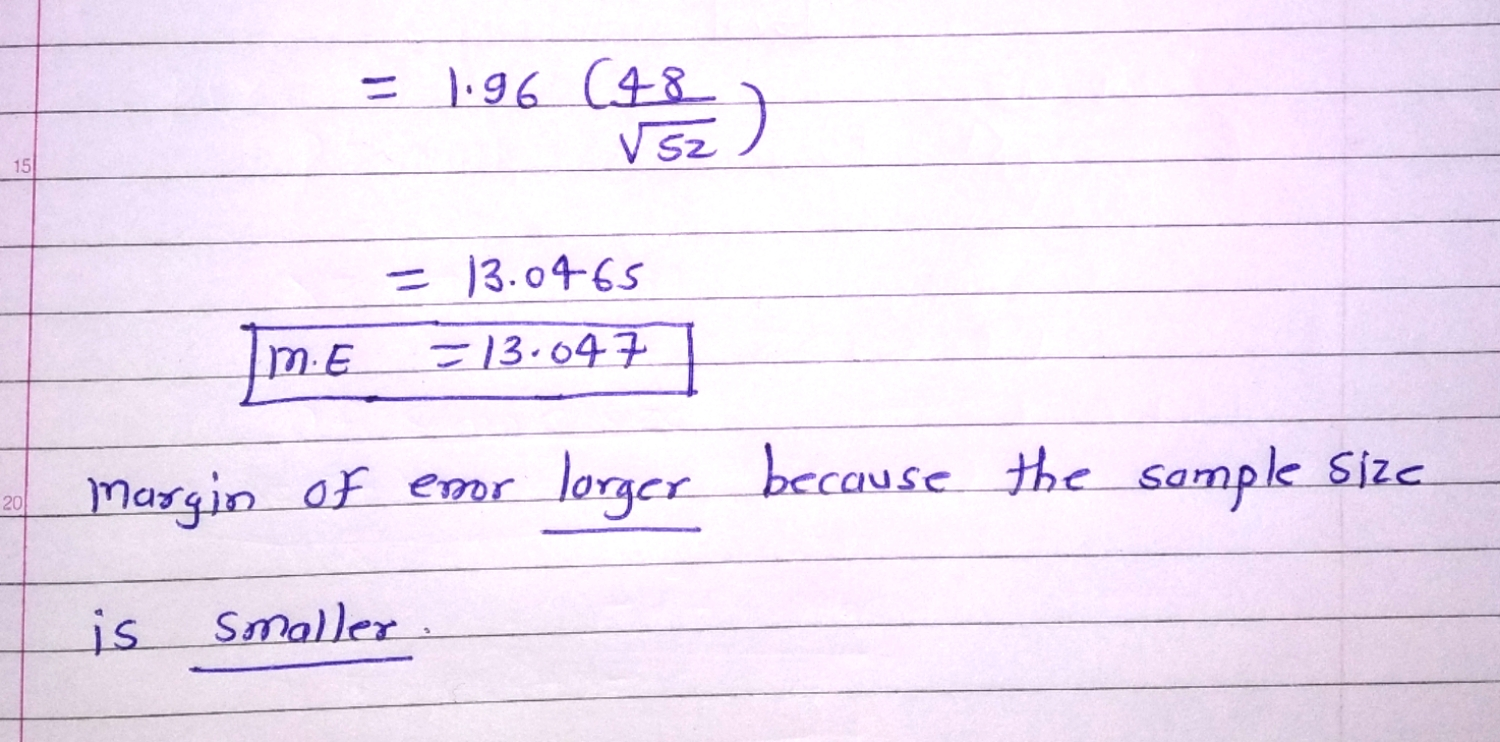#### Earn Coins

Coins can be redeemed for fabulous gifts.

Similar Homework Help Questions
• ### Please solve: Question 3 of 16 (15 points) I Attempt 1 of 1 View question in...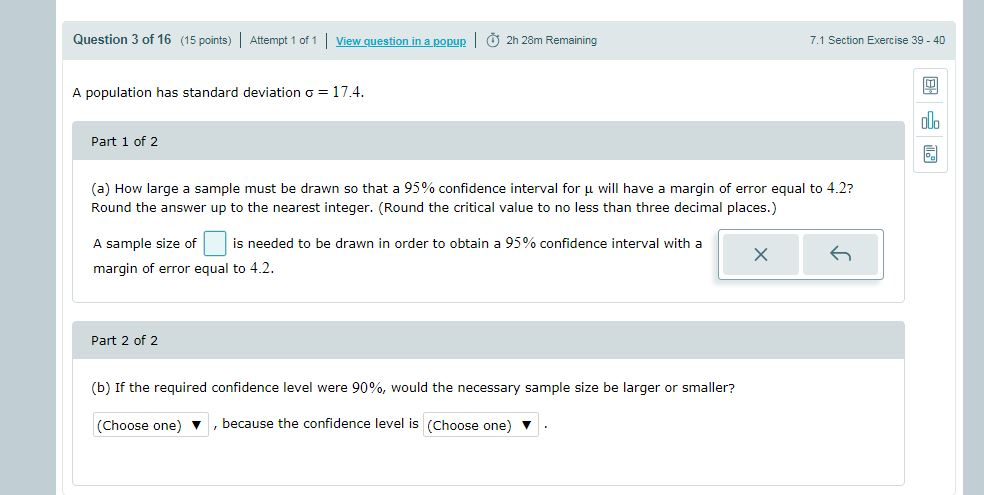Please solve: Question 3 of 16 (15 points) I Attempt 1 of 1 View question in a popup | 卤 2h 28m Remaining 7.1 Section Exercise 39-40 A population has standard deviation 17.4. alo Part 1 of 2 (a) How large a sample must be drawn so that a 95% confidence interval for μ will have a margin of error equal to 4.2? Round the answer up to the nearest integer. (Round the critical value to no less than three...

• ### 7.1 Section Exercise Question 3 of 18 (1 point) Altempt 3 of Unlimited View questlon in...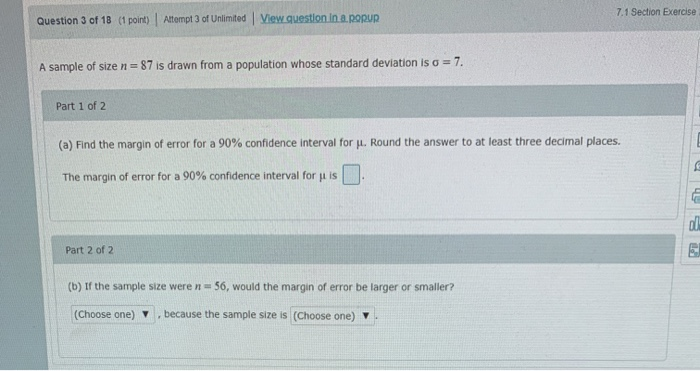7.1 Section Exercise Question 3 of 18 (1 point) Altempt 3 of Unlimited View questlon in a popup 7 A sample of size n 87 is drawn from a population whose standard deviation is a Part 1 of 2 (a) Find the margin of error for a 90% confidence interval for . Round the answer to at least three decimal places. The margin of error for a 90% confidence interval for u is Part 2 of 2 (b) If the...

• ### Part 2 of 2 Lager or smaller.... because the sample size is smaller or larger Question...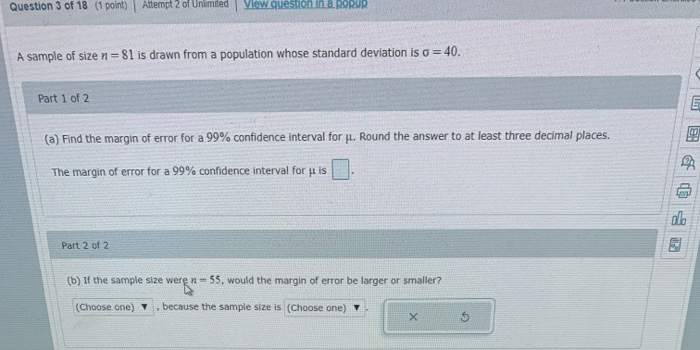Part 2 of 2 Lager or smaller.... because the sample size is smaller or larger Question 3 of 18 (1 point) Attempt 2 of Unlimited View question in a RORUR A sample of size n=81 is drawn from a population whose standard deviation is o = 40. Part 1 of 2 (a) Find the margin of error for a 99% confidence interval for u. Round the answer to at least three decimal places. The margin of error for a 99%...

• ### Question 1 of 13 (1 point) Attempt 1 of Unlimited View question in a popup 8.1...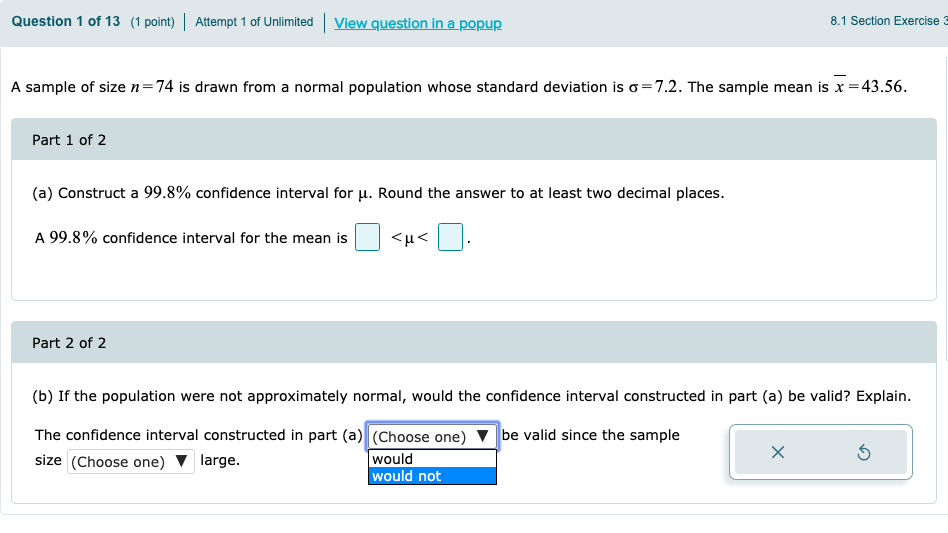Question 1 of 13 (1 point) Attempt 1 of Unlimited View question in a popup 8.1 Section Exercise A sample of size n=74 is drawn from a normal population whose standard deviation is o=7.2. The sample mean is x =43.56. Part 1 of 2 (a) Construct a 99.8% confidence interval for u. Round the answer to at least two decimal places. A 99.8% confidence interval for the mean is <u< . Part 2 of 2 (b) If the population were...

• ### A sample of size n=95 is drawn from a population whose standard deviation iso = 38....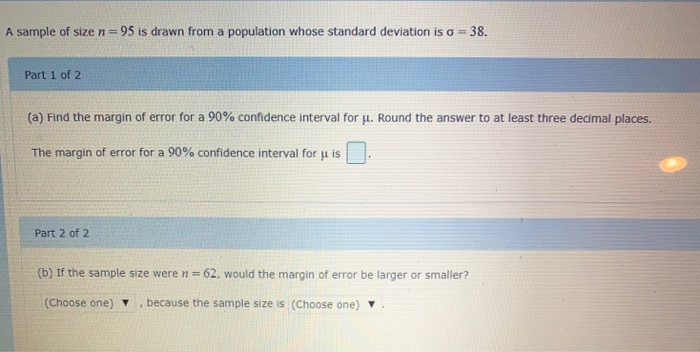A sample of size n=95 is drawn from a population whose standard deviation iso = 38. Part 1 of 2 (a) Find the margin of error for a 90% confidence interval for u. Round the answer to at least three decimal places. The margin of error for a 90% confidence interval for p is Part 2 of 2 (b) If the sample size were n = 62, would the margin of error be larger or smaller? (Choose one) , because...

• ### Question 4 of 18 (1 point) Attempt 2 of Unlimited View question. In a popuR! 7.1 Section Exercise 37-38 A sample...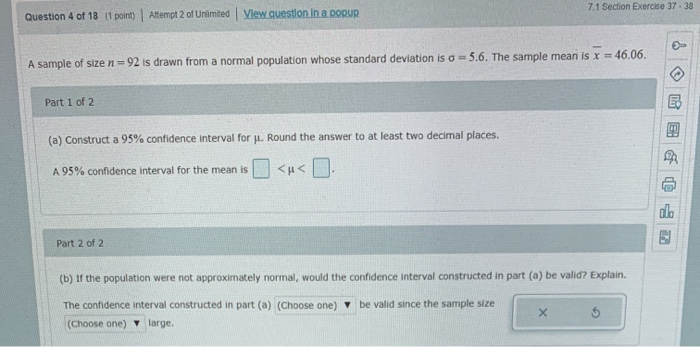Question 4 of 18 (1 point) Attempt 2 of Unlimited View question. In a popuR! 7.1 Section Exercise 37-38 A sample of size n = 92 is drawn from a normal population whose standard deviation is a = 5.6. The sample mean is x = 46.06. Part 1 of 2 (a) Construct a 95% confidence interval for Jl. Round the answer to at least two decimal places. A 95% confidence interval for the mean is <u< . Part 2 of...

• ### A population has standard deviation o-17.3. al. Part 1 of 2 1 (a) How large a...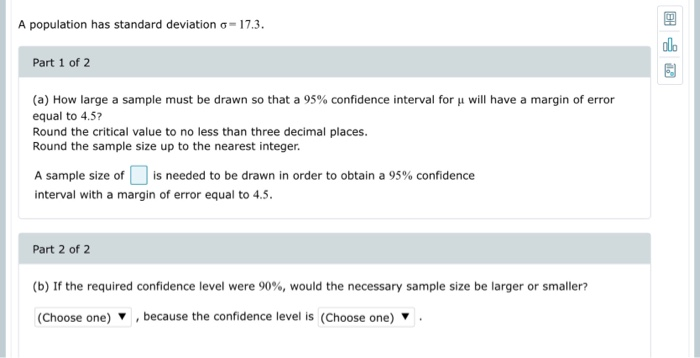A population has standard deviation o-17.3. al. Part 1 of 2 1 (a) How large a sample must be drawn so that a 95% confidence interval for u will have a margin of error equal to 4.5? Round the critical value to no less than three decimal places. Round the sample size up to the nearest integer. A sample size of is needed to be drawn in order to obtain a 95% confidence interval with a margin of error equal...

• ### A sample of size π=95 is drawn from a population whose standard deviation is σ=27.

A sample of size π=95 is drawn from a population whose standard deviation is σ=27. Part 1 of 2 (a) Find the margin of error for a 99% confidence interval for H. Round the answer to at least three decimal places. The margin of error for a 99% confidence interval for u is _______ . Part 2 of 2 (b) If the confidence level were 90%, would the margin of error be larger or smaller?

• ### A population has standard deviation - 17.3. Part 1 of 2 (a) How large a sample...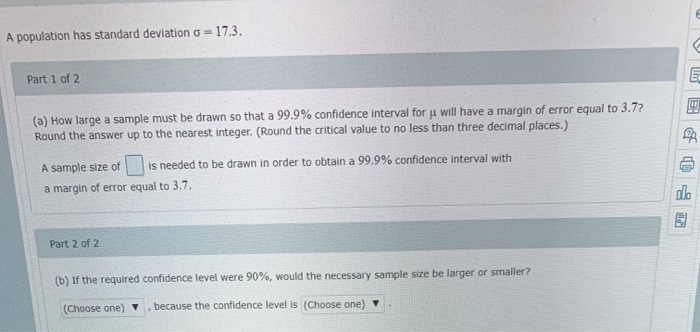A population has standard deviation - 17.3. Part 1 of 2 (a) How large a sample must be drawn so that a 99.9% confidence interval for ji will have a margin of error equal to 3.7? Round the answer up to the nearest integer. (Round the critical value to no less than three decimal places.) A sample size of is needed to be drawn in order to obtain a 99.9% confidence interval with a margin of error equal to 3.7....

• ### Question 7 of 22 (1 point) Attempt 1 of Unlimited View question in a pour 82...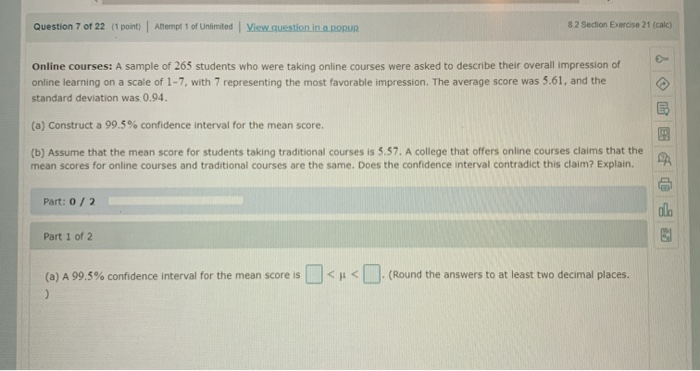Question 7 of 22 (1 point) Attempt 1 of Unlimited View question in a pour 82 Section Exercise 21 (cale) Online courses: A sample of 265 students who were taking online courses were asked to describe their overall impression of online learning on a scale of 1-7, with 7 representing the most favorable impression. The average score was 5.61, and the standard deviation was 0.94. (a) Construct a 99.5% confidence interval for the mean score. (b) Assume that the mean...